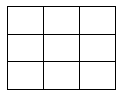# The wonders of the 3 by 3 square (Part 2)

Two variations to explore of the Magic Square of order 3 are given below.

### 1. Product-magic squares.

These are obtained by choosing a set of numbers to be placed into a 3 by 3 grid, see Fig 1, so that the products of each 3 squares in a row, a column, or a diagonal are always the same. The challenge is greater because we need to find the numbers to use, the set of integers 1-9 is not going to lead to a solution.

#### Fig 1

One type of solution can be generated from the traditional magic square by using the laws of indices (choosing a base). The entries can, with appropriate choices, all be integer values. It’s quite a nice activity once the laws of indices have been learned.
Another could be found by finding numbers with exactly 9 distinct factors, and allocating them carefully in the boxes. Is it obvious that such numbers must be square numbers? A useful discussion can be had about why all square numbers have an odd number of distinct factors. This set of solutions is also most likely to lead to using integers.
One further set of solutions could be developed by placing a 1 into the centre square, and perhaps using a, b and their powers in the other squares, and applying the rules. And then substituting chosen numbers for a and b.
It is significantly harder to solve product magic squares of order 4 (except by approach 1 above, based on indices).

### 2. Antimagic squares

These are found by returning to the use of just the digits 1 to 9, but distributing them in a 3 by 3 grid, as in Fig 2 below, so that for each of the 8 possible directions, the total of three consecutive boxes is different.Fig 2

A rather different strategy to any proposed earlier is now needed. There are actually rather a lot of distinct solutions, including some in a logical path.
A mathematically nice result, worth bearing in mind in teaching and learning mathematics, is that once one solution is found, others can be easily found; in this case, by replacing each entry with its tens-complement (the number that makes it up to 10).
The ideas above are very suitable for exploring with Year 7 upwards, others may need more selective application. For learners skillful with coding, some of the above challenges are useful practice.

By Bob Vertes - a semi-retired Mathematics teacher and teacher educator.